NCERT Exemplar - MCQ

Chapter 7 Class 10 Coordinate Geometry
Serial order wise

## (A) 5   (B) 12  (C) 11   (D) 7 + √5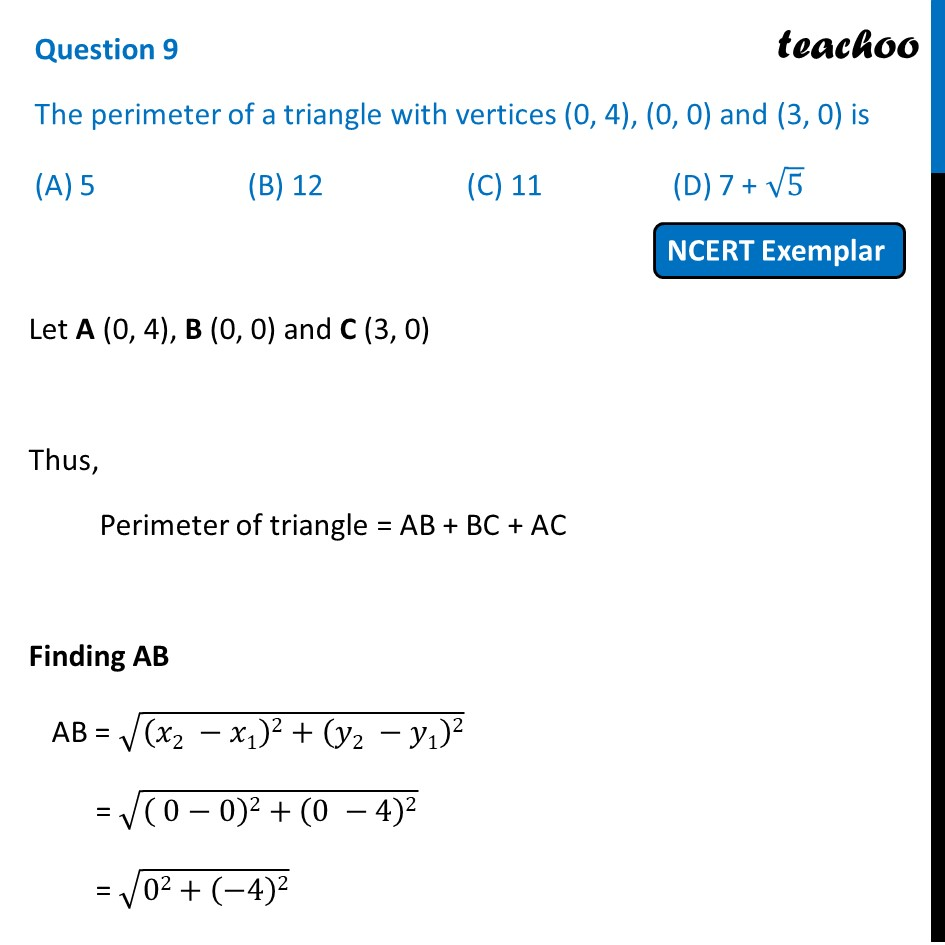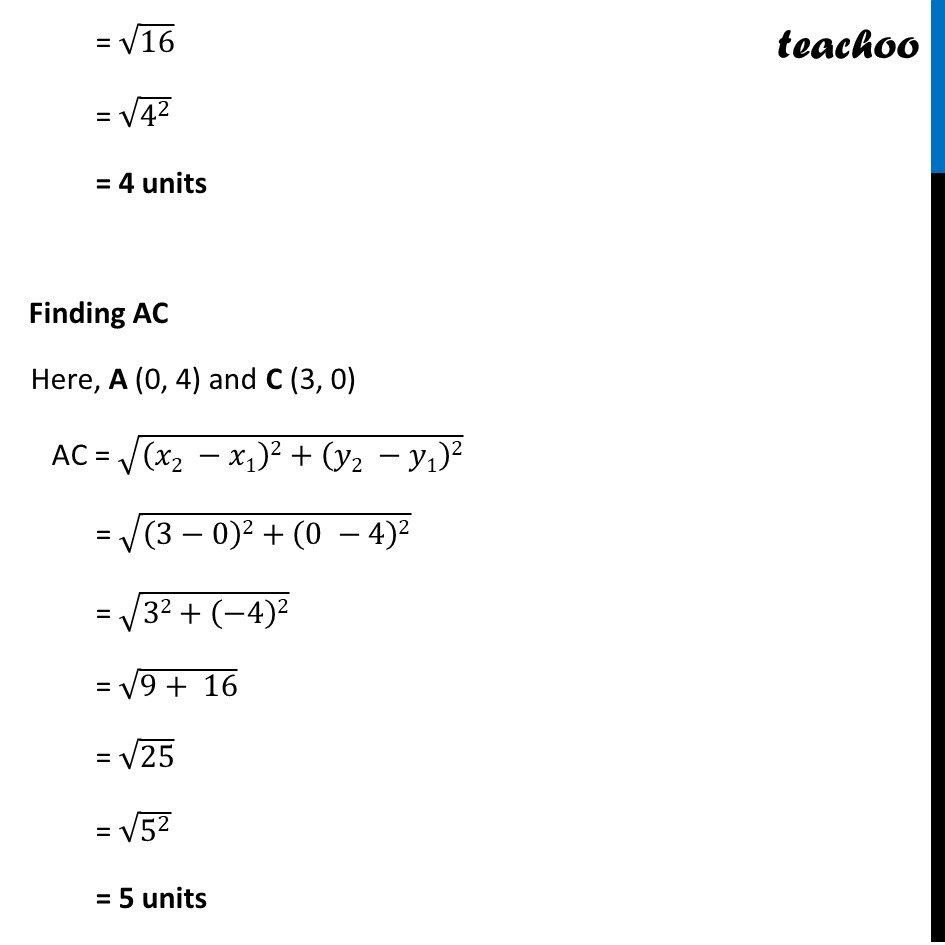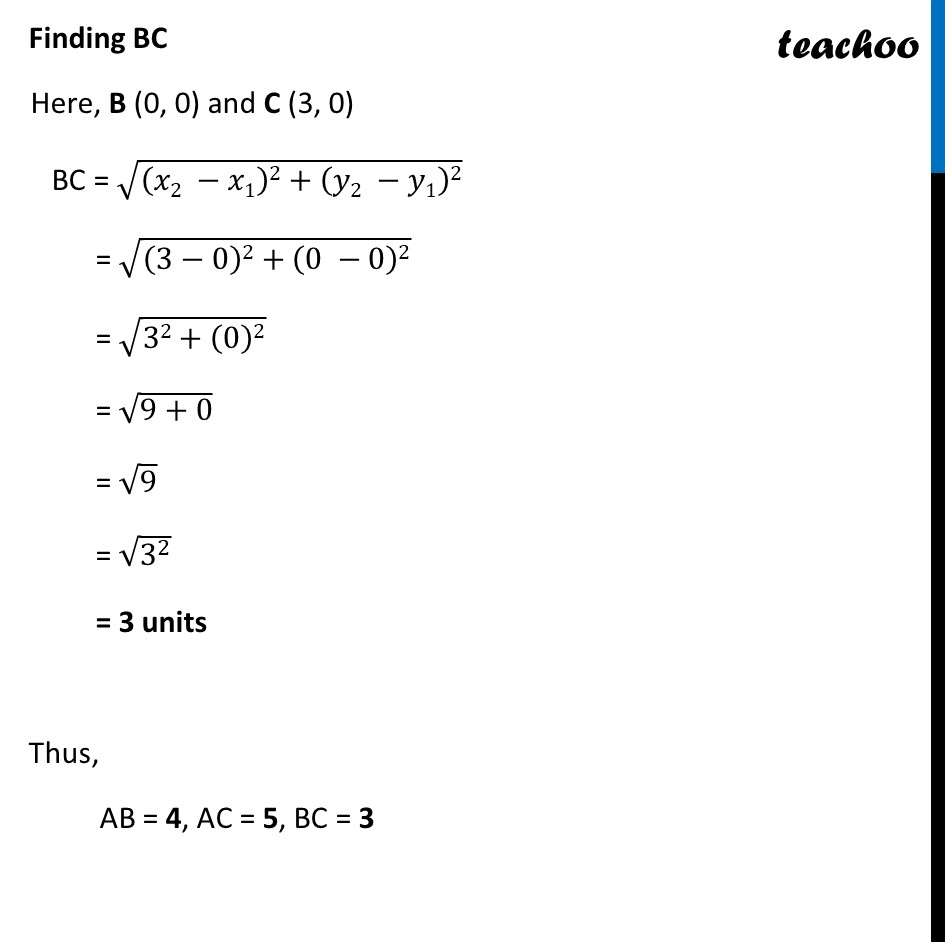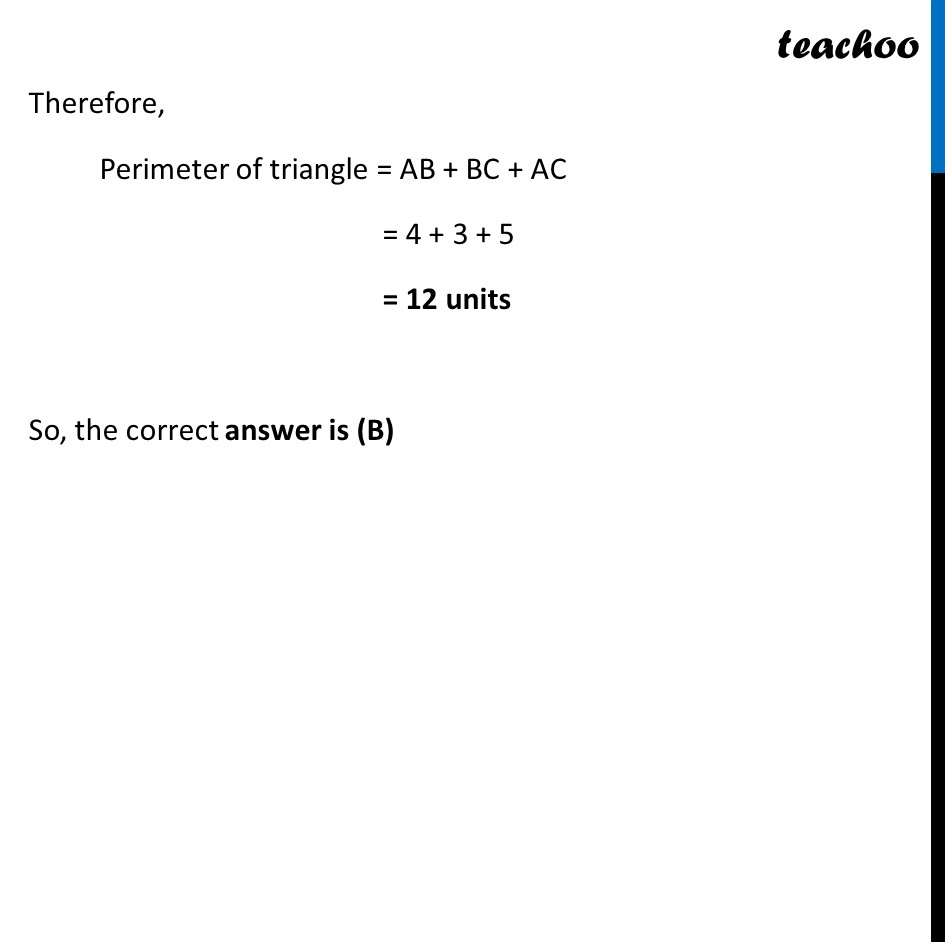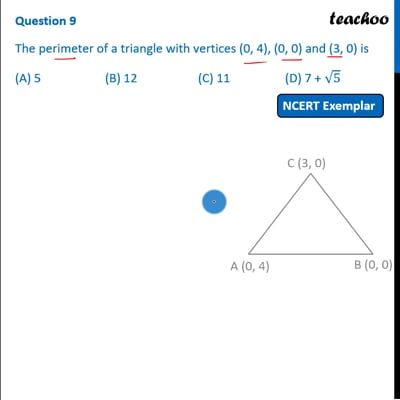This video is only available for Teachoo black users

Introducing your new favourite teacher - Teachoo Black, at only ₹83 per month

### Transcript

Question 9 The perimeter of a triangle with vertices (0, 4), (0, 0) and (3, 0) is (A) 5 (B) 12 (C) 11 (D) 7 + √5 Let A (0, 4), B (0, 0) and C (3, 0) Thus, Perimeter of triangle = AB + BC + AC Finding AB AB = √((𝑥2 −𝑥1)2+(𝑦2 −𝑦1)2) = √(( 0−0)2+(0 −4)2) = √(02+(−4)2) = √16 = √(4^2 ) = 4 units Finding AC Here, A (0, 4) and C (3, 0) AC = √((𝑥2 −𝑥1)2+(𝑦2 −𝑦1)2) = √((3−0)2+(0 −4)2) = √(32+(−4)2) = √(9+ 16) = √25 = √(5^2 ) = 5 units Finding BC Here, B (0, 0) and C (3, 0) BC = √((𝑥2 −𝑥1)2+(𝑦2 −𝑦1)2) = √((3−0)2+(0 −0)2) = √(32+(0)2) = √(9+0) = √9 = √(3^2 ) = 3 units Thus, AB = 4, AC = 5, BC = 3 Therefore, Perimeter of triangle = AB + BC + AC = 4 + 3 + 5 = 12 units So, the correct answer is (B)